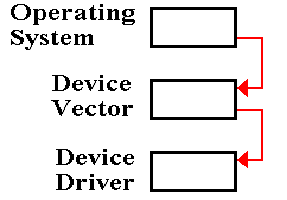Here we review the material from the previous lecture and give other examples.

Modes of interest here span quite a few architectures and might use inconsistent notation.

We begin with the idea of an Effective Address, often denoted “EA” or “E.A.”  This basic idea facilitates understanding the function of any given operation.

Consider two basic operators: Load from memory and Store to memory
¬ M[EA]
Store Register to Memory:             M[EA]
¬ (R)

By definition of the term “Effective Address”, all memory references use that address when accessing memory.  M[EA] denotes the contents of the effective address.

Understanding addressing modes then becomes equivalent to understanding how to calculate the Effective Address.

We begin with a simple mode, called “Direct Address” and then move to a few addressing modes for which the term “Effective Address” is not appropriate.

We then move to a series of increasingly complex addressing modes, ending with a few actually used on commercial machines.

We now consider how to calculate an effective address, given the ISA instruction.

We begin with the format of a generic instruction referencing memory.  The standard format includes an Address Field, which is used in many address calculations.  Often it is the Effective Address, but again can be just part of that address.

The Boz–5 instruction format is as follows.

 Bits 31 – 27 26 25 – 23 22 – 20 19 – 0 Use Five–bit Opcode Indirect Bit Destination or Source Register Index Register Address Field

In general, the only parts of the instruction that are used in computing the EA are

the Index Register  this contains the register used as “array index”.
If this field is 0, indexing is not used.

the Indirect Bit       this is used for Indirect and Indexed–Indirect addressing.
If this is 1, indirect addressing is used; otherwise not.

Instructions that do not use an address field conventionally have that field set to 0.

Before discussing the idea of an Effective Address, let’s discuss instructions
for which the idea of an EA is not appropriate.

No argument:                          HLT                                // Stop the computer.

Immediate Argument:            LDI %R3, 2                     // Load the value 2 into R3.

ADDI %R4, %R4, 1       // Increment R4

98% of Load Immediate and Add Immediate use the values – 1, 0, and + 1.

Register–To–Register:           ADD %R3, %R1, %R2   // R3 = R1 + R2

SUB %R3, %R1, %R2   // R3 = R1 – R2

To clarify the difference between direct and immediate addressing, consider these:

LDI      %R5, 400            // Load register R5 with the value 400; R5 ¬ 400.

LDR    %R5, 400            // Load register R5 with the contents of memory
// location 400; R5
¬ M.  EA = 400.

NOTE: Many of my examples use hexadecimal notation; this example is ambiguous.

 Opcode Registers Mode Flags Address Field

In each of direct and indirect addressing, the computation is simple:
Just copy the address field itself.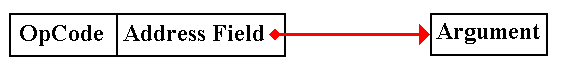In indirect addressing, the address field contains the address of a pointer to the argument.  Put another way, the contents of the memory indicated by the address field contain the address of the argument.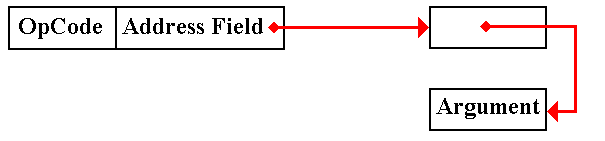Consider the following address map as an example for our addressing modes.

Z represents address 0x0A.  Z == 0x0A and M[Z] = 5.

 Address 5 6 7 8 9 A B C D E F 10 11 Contents 11 32 2C 1E 56 5 7A 10 3 F E D 8

Effective Address:          EA = Z            Here EA = 0x0A.

Effect:                             R1 ¬ M[Z]     Here R1 ¬ M[0x0A] or R1 = 5.

Effective Address:          EA = M[Z]      Here EA = M[0x0A] = 5

Effect:                             R1 ¬ M[EA] = M [ M[Z] ]
Here R1
¬ M[0x05] or R1 = 11.

NOTE:    The complexity of notation such as “R1 ¬ M[ M[X] ]” is one reason we use

This is used to support array addressing in all computers.  Languages such as C and
C++ implement this directly using “zero based” arrays.

In this mode, the contents of a general–purpose register are used as an index.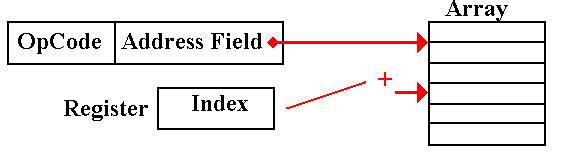EA = (Address Field) + (Register)

Although we expect the index to be smaller than the value of the address, such considerations are not profitable.

The contents of the address field should be considered as having a different data type from those of the register, which holds a signed integer.

LDR %R1, Z, 3     // Z indexed by the general–purpose register R3.

Z represents address 0x0A.  Z == 0x0A and M[Z] = 5.  (R3) = = 4

 Address 5 6 7 8 9 A B C D E F 10 11 Contents 11 32 2C 1E 56 5 7A 10 3 F E D 8

Effective address: EA = Z + (%R3)     Here EA = 0x0A + 4 = 0x0E

Effect:                    R1 ¬ M[EA] = M[0x0E] or R1 = F

In this, the most common, variant of indexed addressing we see Z as an array.
The first five elements of the array are placed in memory as follows:

 Memory Map 0x0A 0x0B 0x0C 0x0D 0x0E Contents: Z Z Z Z Z

Consider the use of general purpose register %R0 as an index register.

LDR %R2, Z, 0

There are two possible interpretations of this instruction.

1.   Do not use indexing in computation of this address.  The effective address is
simply EA = Z and the mode is really direct addressing.

2.   Register R0 is identically 0; %R0 º 0.  Use this value to calculate the effective
address, EA = Z + (%R0) = Z + 0 = Z.  The mode is the same as direct addressing.

OUR MOTTO:     If it acts identically to direct addressing, it is direct addressing.

We shall use this fact to simplify the design of the control unit.

LDR %R4, Z, 7     // Address Z indexed by R7

But suppose Z = 0.  The format of the machine language instruction is:

 31 30 29 28 27 26 25 24 23 22 21 20 19 – 0 0 1 1 0 0 0 4 7 0x00000

The effective address is EA = Z + (%R7) = 0 + (%R7).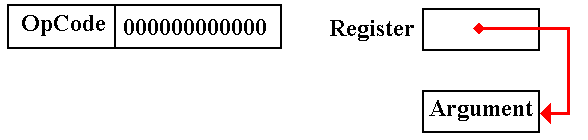This is quite similar to indexed addressing, so much so that it seems redundant.

This mode arises from an entirely different consideration.

Indexed addressing basically supports the use of arrays.

Based addressing supports the Operating System in partitioning the user address space.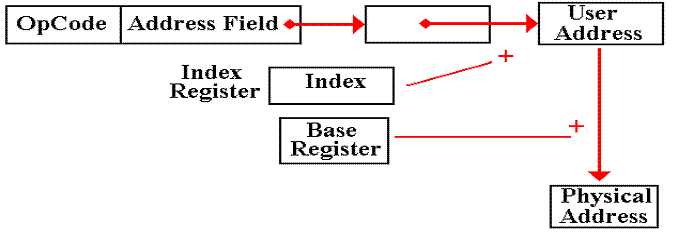The Boz–5 uses the page number in the PSR as a base register.

This is a combination of both modes.  Question: Which is done first?

Pre–Indexed Indirect:  The indexing is done first.  We have an array of pointers.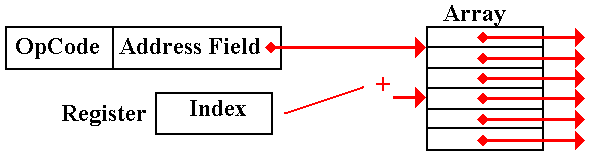Post–Indexed Indirect:  The indirect is done first.  We have a pointer to an array.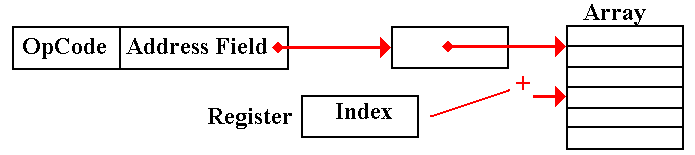Let Z refer to address 0xA, so that M[Z] = = 0x05.  Let (R3) = 0x07.

 Address 5 6 7 8 9 A B C D E F 10 11 Contents 11 32 2C 1E 56 5 7A 9 3 F E D 8

Pre–Indexed Indirect:  LDR %R1, *Z, 3

Effective address    EA = M[ Z + (R3) ] = M[0xA + 0x7]
= M[ 0x11] = 0x08.

Effect                      R1¬ M[ M[Z + (R3)] ] or R1 ¬ M[0x08] or R1 ¬ 1E

Post–Indexed Indirect: LDR %R1, *Z, 3

Effective address    EA   = M[ M[Z] + (R3) ] = M[ M[0x0A] + 0x07]
= M[0x05 + 0x07] = M[0x0C] = 0x09.

Effect                      R1 ¬ M[0x09] or R1 ¬ 0x56

Again, consider the use of general–purpose register R0 as an index register.

Remember that this register is set identically to 0; %R0 º 0.

Pre–Indexed Indirect:  LDR %R1, *Z, 0

Effective address    EA = M[ Z + (R0) ] = M[Z]

Effect                      R1¬ M[ M[Z] ].

But this is just the indirect addressing, discussed above.

Again, this feature will be used to simplify the control unit.

We now come to a more unusual variant of pre–indexed indirect addressing.

Suppose the address part is 0, Z = 0.

LDR %R4, *Z, 7   // Address Z indexed by R7

 31 30 29 28 27 26 25 24 23 22 21 20 19 – 0 0 1 1 0 0 1 4 7 0x00000

The Effective Address is a bit unusual

Effective address    EA = M[ Z + (R7) ] = M[0 + (R7)] = M[(R7)]

Effect                      R1¬ M[ M[(R7)] ]

R7 contains the address of a pointer.  R7 is a pointer to a pointer.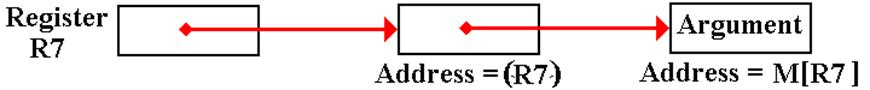We would have to make the control unit more complex to remove this addressing mode.

We now simplify our control unit by introducing a new register convention.

As is common among many modern computers, we define a register zero, called R0
and demand that it be identically 0.  Thus R0 º 0.

Stores to register R0 are ignored.  This register cannot be changed.

Consider two instructions
Load        X, (R0)            EA = X + (R0) = X         Really direct addressing.

Consider a typical address part of an instruction:

 Mode Field Index Register Address Field

There are two options for the Index field.
More complex        If zero, use direct addressing
If not zero, use the register as an index register.

Less complex          Use the given register as an index register for indexed

At the microarchitecture level, we lose no efficiency in always adding the register to form the address.  We gain a great deal by simplifying the address computation.

Suppose a common data structure that is used by a number of programs.
In modern systems, this could be a DLL (Dynamic Linked Library) module.

Each program contains a pointer to this structure, used to access that structure.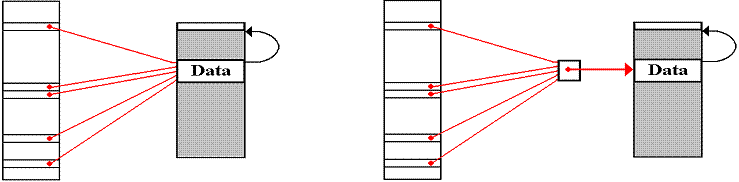Singly indirect addressing, in which the pointer is stored in every program, presents problems when the data block is moved by the memory manager.  Every reference to that block must be found and adjusted.

In doubly indirect addressing, all programs have a pointer to a data structure which itself is a pointer.  When the data block is moved, only this one pointer must be adjusted.

As an extra benefit, this intermediate pointer can be expanded to a data structure containing an ACL (Access Control List) and other descriptors for the data block.
This presents the beginning of a more secure operating system.